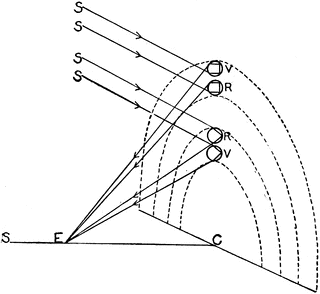# Are rainbows three dimensional? If so, what determines their depth? [duplicate]

I am wondering whether rainbows are three dimensional, and if so, what determine their depth? How to calculate the depth of a rainbow, given its radius?

From what I understand, all rainbows are circular and their radius are determined by the distance between the observer and the water drops. How can one determine the depth based on this information, assuming the depth is non zero?

Edit: As stated in a comment by Solomon Slow, a rainbow is not really an object. My question still applies, the rays of light that make us see a rainbow are coming from droplets. What is the depth extension of these (real) droplets?

• @tttt, it is probably more relevant to ask about how many water droplets are at the appropriate angle to produce a rainbow. Those water droplets don't have a definite depth, but the DO have a definite angle. Jan 21, 2019 at 21:21
• @DavidWhite Yeah, that's the solution to my question. If the could extends infinitely, the depth would be infinite. I did not realize this! I thought there was a finite depth that would depend on the distance between the observer and the drops. Jan 21, 2019 at 21:22
• Duplicate How deep is a rainbow and links therein. Jan 21, 2019 at 21:47A rainbow doesn't strictly have a radius.

First: the obvious. A rainbow isn't a 'circle' but an annulus. The thickness comes from the range of frequencies (colors). However, if we restrict ourselves to one frequency, it's a circle.

No matter where you stand, the rainbow will appear as a circle centered around the antisolar point with an angle of $$\theta_{\rm{crit}}\approx 42^o$$ (note, the image above shows 2 different angles - this is a double rainbow. You can ignore the outer rainbow.). If the rainbow was a definite distance $$d$$ from you, it would have a definite radius $$r=d \tan(\theta_{\rm{crit}})$$. But it isn't!

The light which reaches your eye comes from any droplet of water located on a cone that has yourself as the vertex, the direction of sunlight as an axis and an angle of $$\theta_{\rm{crit}}$$. If the rain was confined to a thin sheet perpendicular to the sunlight (an obviously artificial configuration), only then would it's intersection with the cone be a definite circle with a definite radius.

As for determining thickness: roughly speaking a fainter rainbow should correspond to less thickness (fewer droplets contributing to the cone of light).

• I already know all of that, but your sketch is incomplete and does not deal with my question. In your sketch the rainbow is drew on a 2d surface. So your answer basically claims it's as if the light came out of a 2d object. Is that really so? Jan 21, 2019 at 20:28
• And by the way, it certainly has a radius. In your sketch, the CV segment (or CR, it makes almost no difference in a real situation where the rainbow is "far away). Jan 21, 2019 at 20:30
• > "And by the way, it certainly has a radius. In your sketch, the CV segment (or CR, it makes almost no difference in a real situation where the rainbow is "far away)." That's because the sketch is only considering a thin sheet of rain. You can think a 'typical' rain as many thin sheets pressed together. Jan 21, 2019 at 20:33
• Exactly and this is what I want to know. Say the vertical sheets do extend a lot in front/on the back. The rainbow will be formed by only a part of these sheets, not all, I suppose. I want to know how to compute that distance mathematically, given the distance between the observer and the drops. Jan 21, 2019 at 20:37
• I'm not sure I understand. Are you trying to build a picture of where in space the droplets are? As mentioned in the answer, the intensity of the light from the rainbow at a particular angle (azimuthal, not $\theta$ which is constant) should roughly correspond to how many droplets lie on that line, so that gives you a very rough way to estimate the thickness. Other then that, I don't think there's anything you could do. Jan 21, 2019 at 20:47

In theory, if conditions are right, a rainbow can have a depth of several kilometres. But for this you would need the rainclouds to have just the right structure so that enough sunlight could get to the rain that they were producing, and then back to your eye. They would need to form a kind of enormous funnel. So that's not very likely. Also, the back of such a rainbow would contribute little to the spectacle, because the intervening rain would block out most of the reflected light.

In general, I would expect a decent rainbow to be of the order of hundreds of metres deep. This is not based on any scientific measurements, just on an understanding of the geometry involved.

No.

Rainbows aren't objects, so they don't have dimensions in the naïve sense.

Yes.

Rainbows are visible because droplets of water reflect and refract the sunlight into your eyes. The colors are refracted at slightly different angles, approximately at 42°. All droplets that are on a ray originating in your eye will refract the same color into your eye and depending on the current state of the atmosphere, there may be more or less droplets and they will probably be located in a certain volume that could be considered "the rainbow".